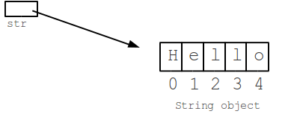# Java Number Of Words In A String | 4 Ways

Java Program To Calculate Number Of Words In A String – In this article, we will brief in on all the methods to calculate the number of words in a string. The following java code has been written in multiple ways, if you have any suggestions or queries do leave a comment about number of words in string.

• Using a Static Method
• Using Scanner Class
• Using Command-Line Arguments
• Using Separate Class

A string is a data type used in programming like an integer or a floating – point but it is used to represent text whenever it is required instead of numerical.As you can see, the string mentioned here is “Hello”. The string consists of 5 characters. All of the 5 characters are represented by a pointer. The location is represented by a pointer, in this case, starting from 0 to 4.

Similarly, other strings also have ideal lengths where the space between the two words is also counted.

Thus, the methods used to find the length of a string in Java programming are as follows

## Number Of Words In A String – Using a Static Method

Static methods in Java are known for beings the methods that work without the need for creating objects of that class.

In here, initially a string is read and stored in  String variable ‘a’.

Then the static method named ‘Length’ is called. In the Length method, an integer variable c is declared which maintains the count of the number of words in the input string.

Every character in the string is checked for a few conditions. First it’s checked whether the index is not out of bound and whether the character at that position in a blankspace.

This is done because one word ends with a space. If it is a space, then it is immediately verified if the character before it is a space as well.

This is done only to ensure that one doesn’t end up counting the same word twice (in case we give 2 or more blankspace while entering a string). When all these conditions are met, then the count (variable c) is incremented by 1.

Another possibility is to encounter a blankspace in the beginning of the string itself, even under that case we increment the count by 1.

Output:

Using Scanner Class

Scanner is a class that is mainly meant to be used for taking inputs at runtime to make it much more user friendly.

This can be used for different primitive data types like int,double,etc.

Even in case of scanner class, the main logic of the program is still the same as the one used in Static method, the only difference here is that, we do not use a separate static method to invoke the main logic. In the main method itself, the logic is written.

Output:

### Words in a String Java – Using Command Line Arguments

Command line arguments in Java are the arguments given in the command line while running the java program.

Similarly here, we can directly pass the string as an argument in command line while running the code.

Since every word is naturally separated by space, by just using the length method we can figure out the number of words in the string we passed.

Not just that, every argument(word) is given a separate index as well, so we can also find the positioning of each word as well.

Output:

Using Separate Class

Another possible way of writing this is, in a separate class. In this case the length method (constructor) is written in a different class consisting of the same logic. Then, in the main class, under the main function we create an object referring to the length class.

When the object is created, automatically the methods and data is the Length class is now usable in the main class as well. So, by calling the length method it automatically goes through the execution of the logic and returns the count of words.

Output:

x

## Java Program to Add Two Matrices – 4 Ways | Programs

Java program to add two matrices – The following Java Code will let you know ...